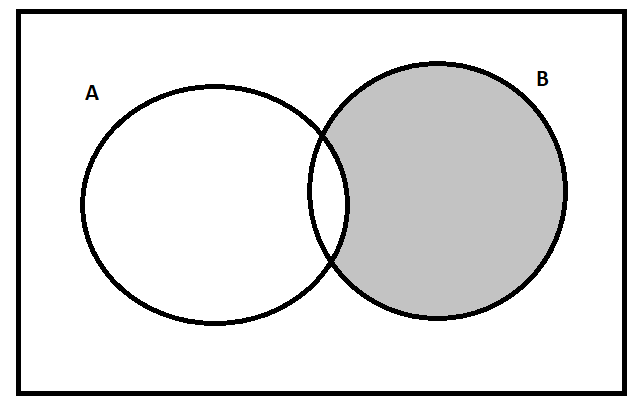Courses
Courses for Kids
Free study material
Free LIVE classes
MoreLIVE
Join Vedantu’s FREE Mastercalss

# Let A = {3, 6, 12, 15, 18, 21}, B = {4, 8, 12, 16, 20}, C = {2, 4, 6, 8, 10, 12, 14, 16} and D = {5, 10, 15, 20}. Find B – A.Verified
361.8k+ views
Hint: In set theory, the subtraction of a set A from a set B is given by the set obtained by removing all the elements of the set A from the set B. Using this concept, we can solve this question.

Before proceeding with the question, we must know the concept that will be required to solve this question.
Let us assume that we are given two sets A and B. In the set theory, the subtraction of the A from the set B which is denoted by B – A is given by the set obtained by removing all the elements of set A from the set B.The shaded part in the above venn diagram represents the set B – A.
In this question, we are given set A = {3, 6, 12, 15, 18, 21} and set B = {4, 8, 12, 16, 20}. We are required to find B – A.
We can clearly observe that in set B, the only element which is also present in set A is 12. So, B – A is given by the set obtained after removing the element 12 from the set B.
$\Rightarrow$ B – A = {4, 8, 16, 20}
Hence, the answer is the set {4, 8, 16, 20}.

Note: There is a possibility that one may remove the elements of set B from the set A instead of removing the elements of set A from the set B to find B – A which will give us an incorrect answer.
Last updated date: 29th Sep 2023
Total views: 361.8k
Views today: 4.61k